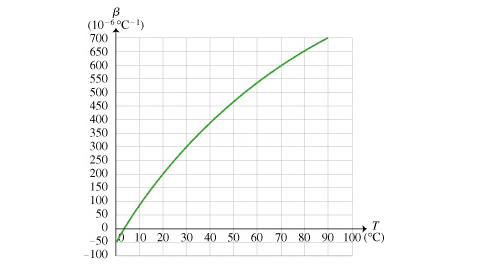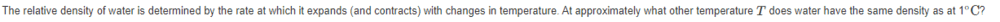# Density of water

nicky670
Homework Statement:: The relative density of water is determined by the rate at which it expands (and contracts) with changes in temperature. At approximately what other temperature T does water have the same density as at 1 ∘C ?
Relevant Equations:: Just looking at graphical and analyzing. But i can't seem to figure out why the answer is 8 degree celsius.Homework Helper
Gold Member
Do you know at what temperature the water's density is highest? It's a bit difficult to read it from the graph.

Gold Member
Hi and welcome to PF
The relevant region of the graph is very tiny but look at what the graph is telling you. At 0°C it is contracting (negative sign for β) by about 50 per degree. Where is it expanding by 50 per degree? What does that say about where the maximum density is and what would the Density / temperature graph look like?
I think it would be perfectly in order for you to Search about the way the density of water changes with temperature, near freezing point and relate that to the graph.
I don't need to give you the actual answer to your question now, do I?

Gold Member
@nicky670 that could be seen as a tricky question to be presented with unless you are familiar with Calculus and the meanings of differentials. It was easy for me / PF because we knew the answer already and it was mainly a matter of reconciling the answer with the graph (reverse process of how you're supposed to do it)

nicky670
Do you know at what temperature the water's density is highest? It's a bit difficult to read it from the graph.
No it is not stated

nicky670
Hi and welcome to PF
The relevant region of the graph is very tiny but look at what the graph is telling you. At 0°C it is contracting (negative sign for β) by about 50 per degree. Where is it expanding by 50 per degree? What does that say about where the maximum density is and what would the Density / temperature graph look like?
I think it would be perfectly in order for you to Search about the way the density of water changes with temperature, near freezing point and relate that to the graph.
I don't need to give you the actual answer to your question now, do I?
will do so and get back to you shortly. Thank you

Homework Helper
Gold Member
No it is not stated
You can look it up or read it off the graph that @berkeman provided, it's 4 oC. That's where the graph you provided crosses the temperature axis. So if the density is highest at 4 oC, what happens to the density if you increase the temperature from 4 oC by 1 degree? What happens to the density if you decrease the temperature from 4 oC by 1 degree?